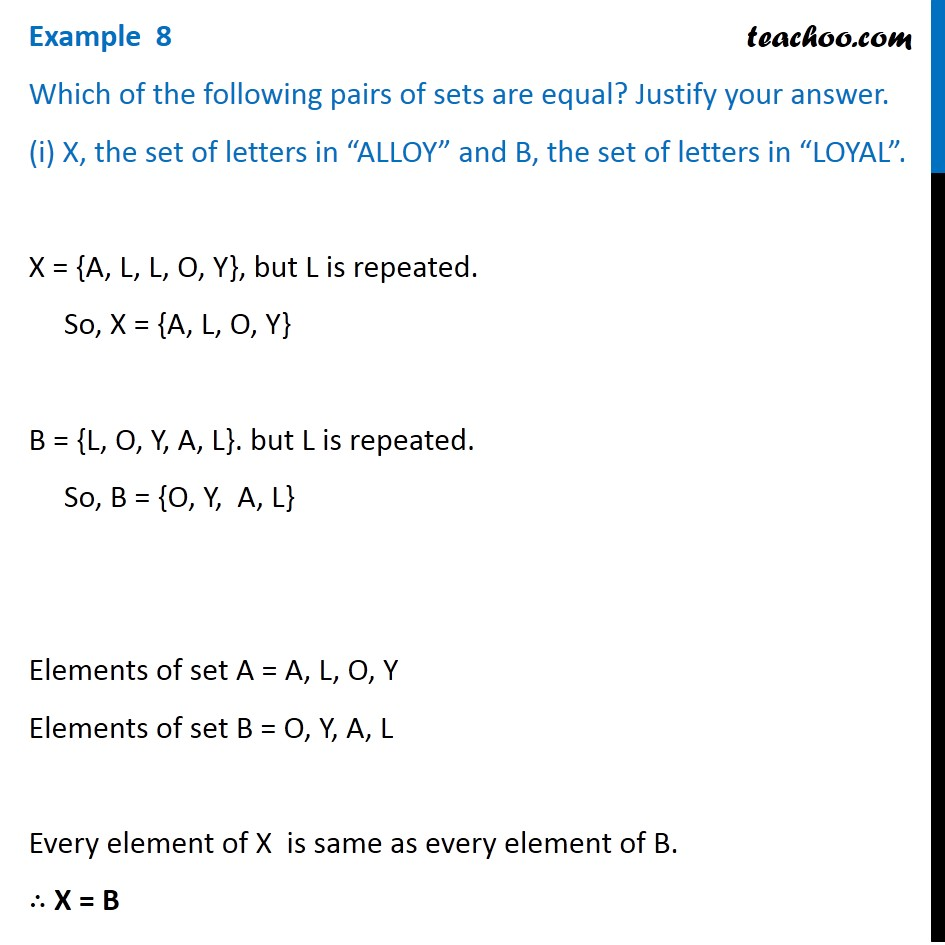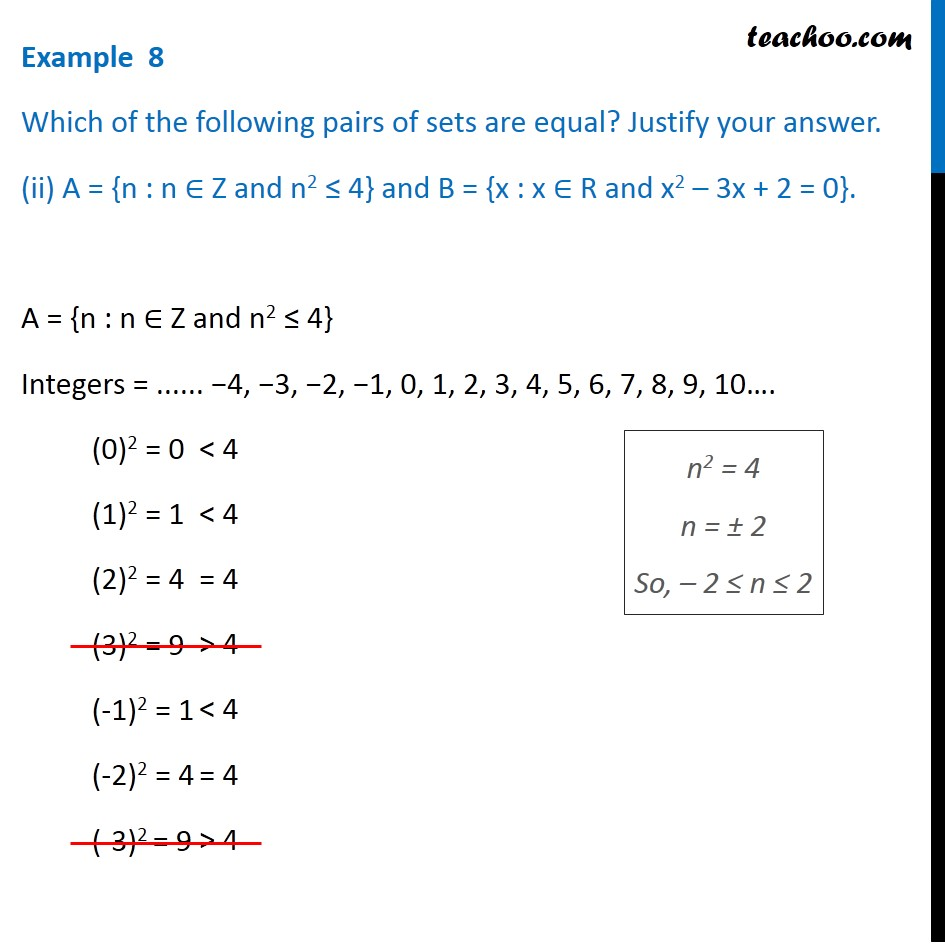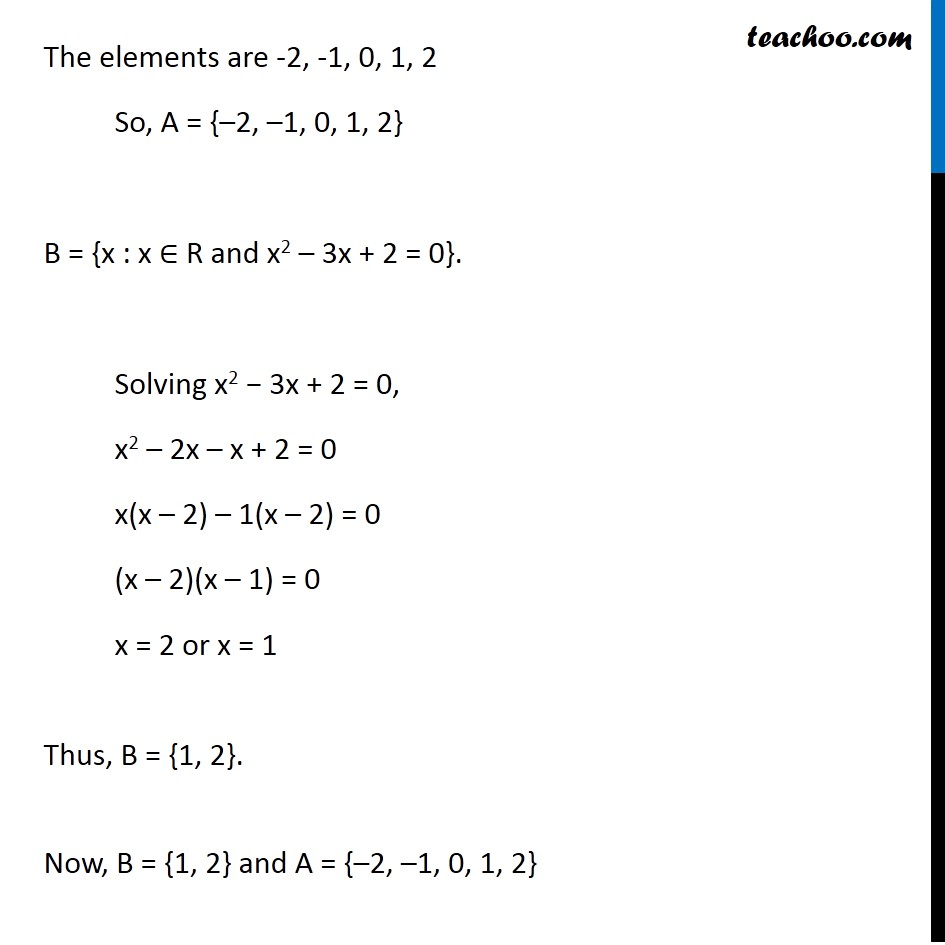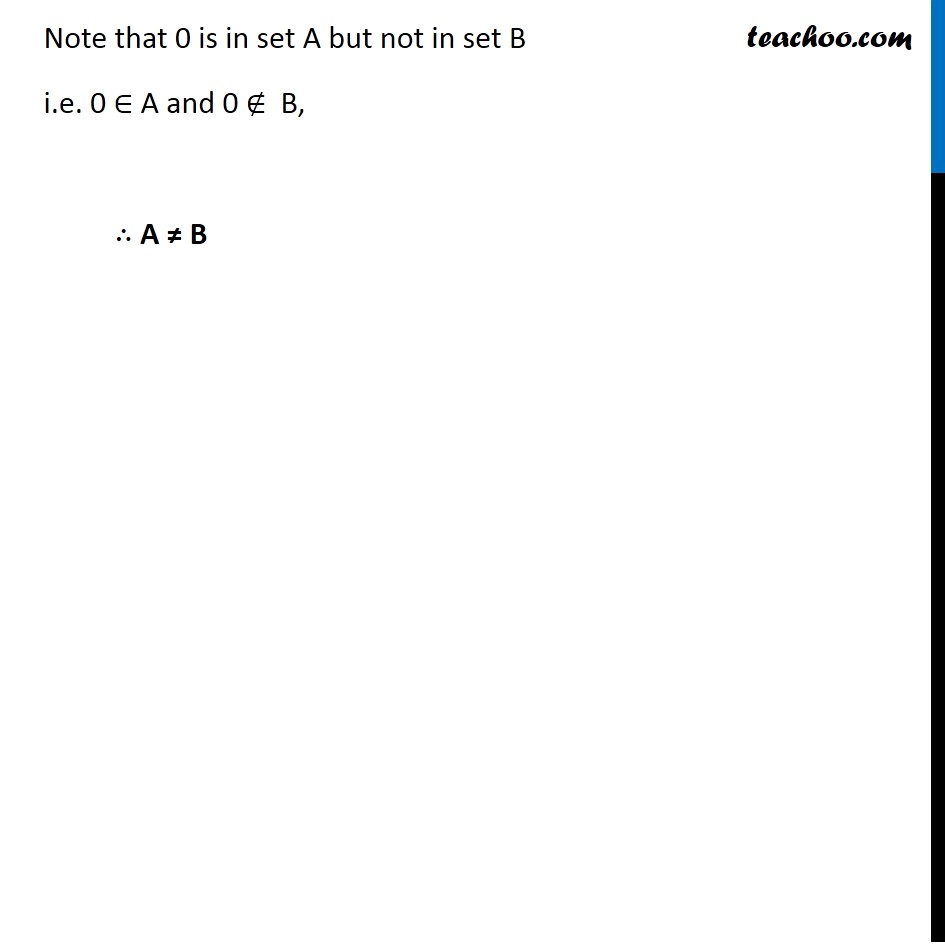1. Chapter 1 Class 11 Sets
2. Concept wise
3. Equal sets

Transcript

Example 8, Which of the following pairs of sets are equal? Justify your answer. (i) X, the set of letters in ALLOY and B, the set of letters in LOYAL . X = {A, L, L, O, Y}, but L is repeated. So, X = {A,L,O,Y} B = {L, O, Y, A, L}. but L is repeated. So, B = {O,Y, A,L} Elements of set A = A, L, O, Y Elements of set B = O, Y, A, L Every element of X is same as every element of B. X = B

Equal sets

About the AuthorDavneet Singh
Davneet Singh is a graduate from Indian Institute of Technology, Kanpur. He has been teaching from the past 10 years. He provides courses for Maths and Science at Teachoo.